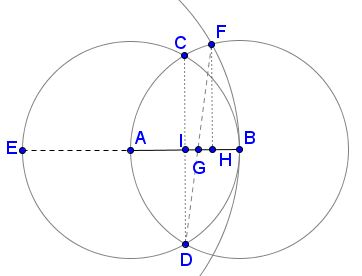# 5-Step Construction of the Golden Ratio, One of Many

In the early 2000s, in a series of papers, K. Hofstetter presented several 5-step construction of the Golden Ratio. In the resulting diagram, Hofstetter observes not one but two occurrences of the Golden Ratio. More recently (30 May, 2016), Tran Quang Hung found in the same diagram additional instances of the famous number.

Hofstetter's construction is this. For a given segment $AB$ of length $1,$ form circles $A(B)$ and $B(A).$ Let $C$ and $D$ be the intersections of $A(B)$ and $B(A).$ Extend $AB$ beyond $A$ to the intersection $E$ with $A(B).$ Draw $E(B)$ and let $F$ be the intersection of $E(B)$ and $B(A)$ farther from $D.$ $DF$ intersects $AB$ in $G.$ $AG:BG = \varphi .$To see why this is so, let $I$ be the intersection of $CD$ and $AB$ and $H$ the foot of perpendicular from $F$ to $AB.$Then

$IG/GH = DI/FH = (\sqrt{3})/2 / (\sqrt{15})/4 = 2/\sqrt{5}.$

It follows that $IG = 2\cdot IH/(\sqrt{5} + 2) = (\sqrt{5} - 2)/2,$ and $AH = 1/2 + IG = (\sqrt{5} - 1)/2.$ This shows that $G$ divides $AB$ in the golden section.Hofstetter also observed another occurrence of the Golden Ratio. If $K\;$ is the intersection of $CF\;$ with the diameter $JB,\;$ then $KA:AB=\varphi:$More recently, Tran Quang Hung posted at the CutTheKnotMath facebook page, practically in the same diagram, additional instances of the Golden Ratio. First, $\displaystyle\frac{AE}{KE}=\varphi.$Also, if $L\;$ is the second intersection of $CF\;$ with circle $E(B),\;$ then $\displaystyle\frac{CK}{KL}=\varphi.\;$ And here's Tran Quang Hung's another observation:If $DN\perp CF,\;$ as shown, and $M=DN\cap B(A),\;$ then $\displaystyle\frac{DM}{MN}=\varphi.$

### References

1. K. Hofstetter, Another 5-step Division of a Segment in the Golden Section, Forum Geometricorum, v 4 (2004), pp. 21-22### Golden Ratio